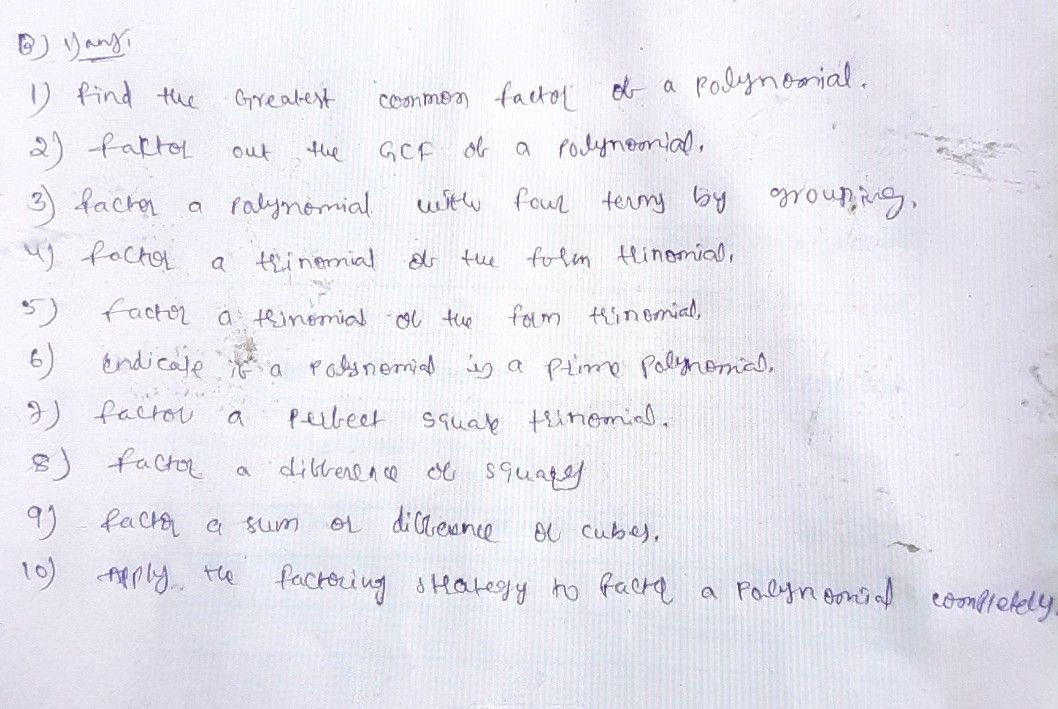Symbol
ProblemB. Answering the following questions. $1.$ How do you factor polynomials? Discuss the mathematics concepts and principles applied when factoring polynomials. $2.$ What new realizations do you have about factoring polynomials? $-0^{-}$ Would you connect this to real life? How would $5$ you use this in $=2i.\square z$ decisions?